# Mathematics 1010 online

## The Real Number Line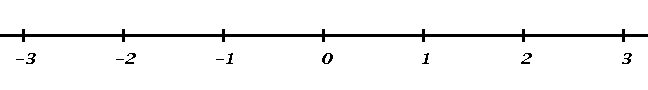### The Real Number Line

The real number system can be visualized as a horizontal line that extends from a special point called the Origin in both directions towards infinity. Also associated with the line is a unit of length. The origin corresponds to the number 0. A positive number x corresponds to a point x units away from the origin to the right, and a negative number -x corresponds to a point on the line x units away from the origin to the left. All of this is illustrated in the above Figure.

We say that a number x is greater than a number y, in symbols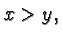if on the real number line x lies to the right of y. If we want to include the possibility that x is actually equal to y then we say that x is greater than or equal to y, and we denote this fact by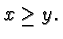For example,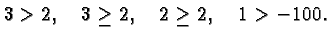Similarly, we say that x is less than y, in symbols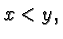if x lies to the left of y, and x is less than or equal to y, in symbols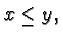if x may be equal to y but no greater than y. For example,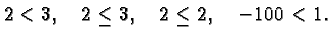Now get ready for a bit of convoluted logic that often confuses students in Math 1010.

A true statement such as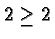might surprise you since it's obviously not true that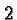is greater than. Things may be clearer if you pronounce the statement in words:is greater than or equal to. The operative word here is or. A statementis true in any of the following three cases:
•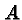is true andis false
•is true andis false
•andare both true.becauseequals. That's one of the two parts of the meaning of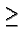. It may be tempting but would be wrong (false) to make the following statement:is false sinceis not greater than.

Here is another example. It is a true statement that Napoleon was a man or a woman because he was a man. The fact that he was not a woman does not make the statement false.

The utility of the symbolis more apparent when we don't know the particular relationship. For example we may know that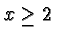, even if we don't know whether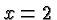or. Indeed, one major technique in mathematics to establish equality is to show separately that one quantity is greater than or equal to the other, and also that it is less than or equal to the other.

The absolute valueof a real number x is its distance from the origin. If x is positive thenand if x is negative then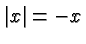. (Remember that the negative of a negative number is positive.) So, for example,.

Slightly more subtle facts that you may want to ponder is that for all real numbers x and y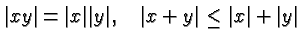and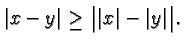Hint: check this for all possible sign combinations of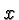and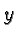.

The distance between two real numbers x and y is the absolute value of their difference. For example, the distance between 3 and 5 is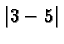(i.e., 2) which makes perfect sense if you visualize this on the real number line. A more complicated example is provided by the distance d between 3 and -2. We have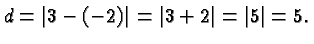Again, this is consistent with the usual notation of distance a long a line.